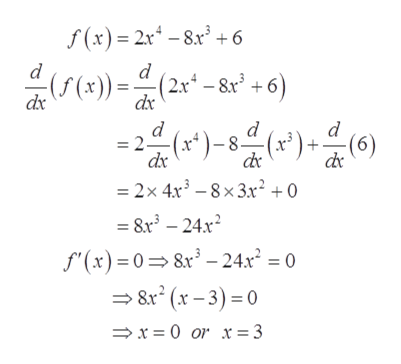# Find all relative extrema of the function 2x^4 - 8x^3 + 6. Use the Second Derivative Test where applicable.

Question
2 views

Find all relative extrema of the function 2x^4 - 8x^3 + 6. Use the Second Derivative Test where applicable.

check_circle

Step 1

To determine the all relative extrema of the function.

Step 2

Given:

Step 3

Calculate the critical points of the function ...help_outlineImage Transcriptionclosef (x) = 2x* – 8.r³ + 6 - &r* +6) -(f (x)) = (2x* – &x³ +6) (x) = dx (x*)-8 = 2(*)-8-(x*) + (6) de = 2x 4x – 8× 3x² + 0 = 8x – 24.x? S"(x) = 0=8x* – 24x² = 0 = 8x (x – 3) = 0 → x = 0 or x = 3 fullscreen

### Want to see the full answer?

See Solution

#### Want to see this answer and more?

Solutions are written by subject experts who are available 24/7. Questions are typically answered within 1 hour.*

See Solution
*Response times may vary by subject and question.
Tagged in

### Functions